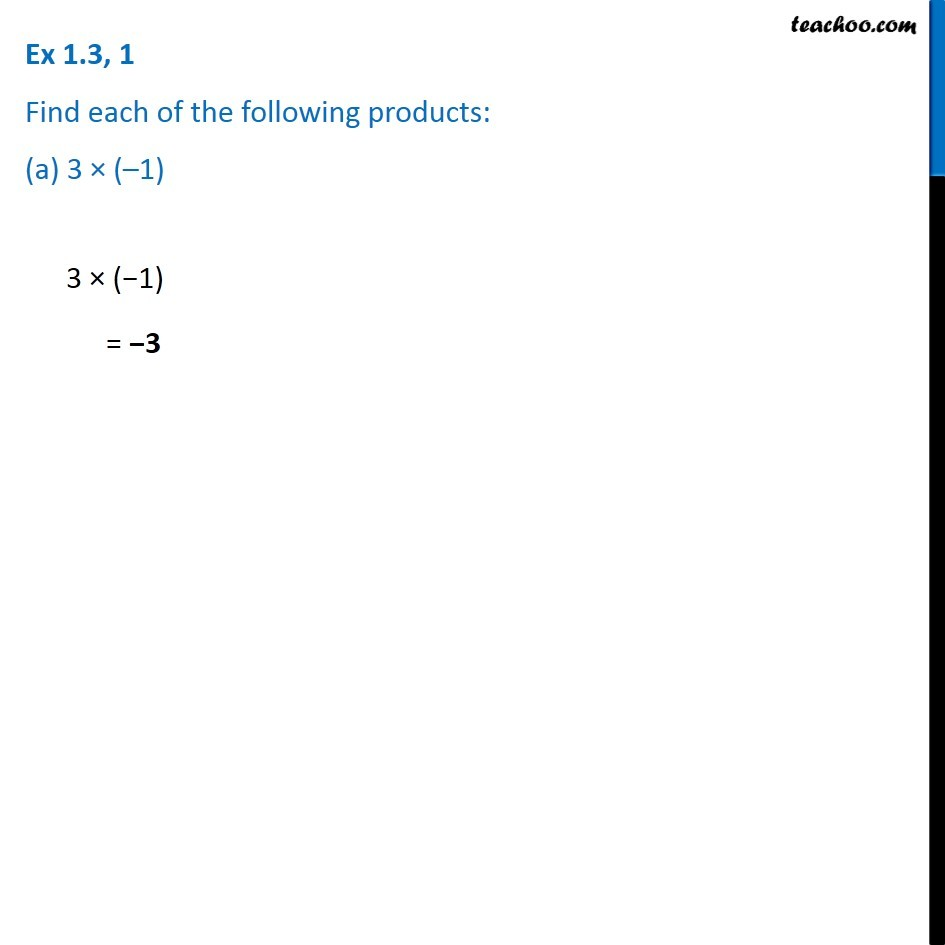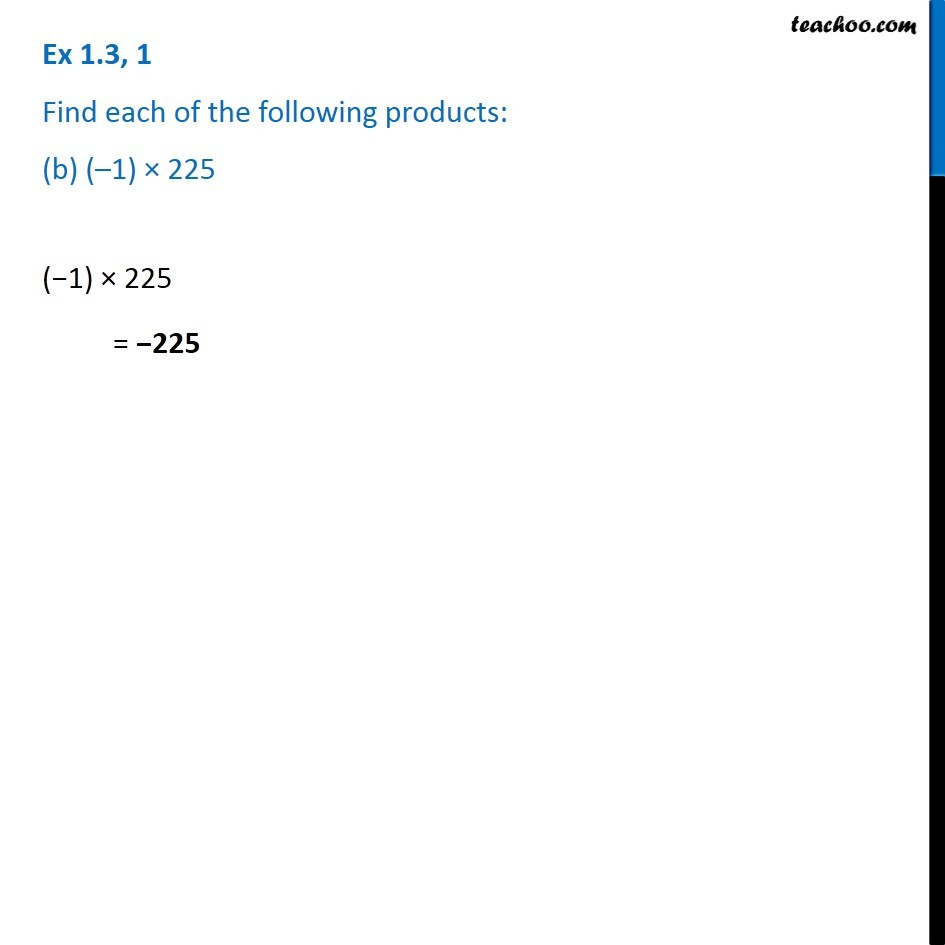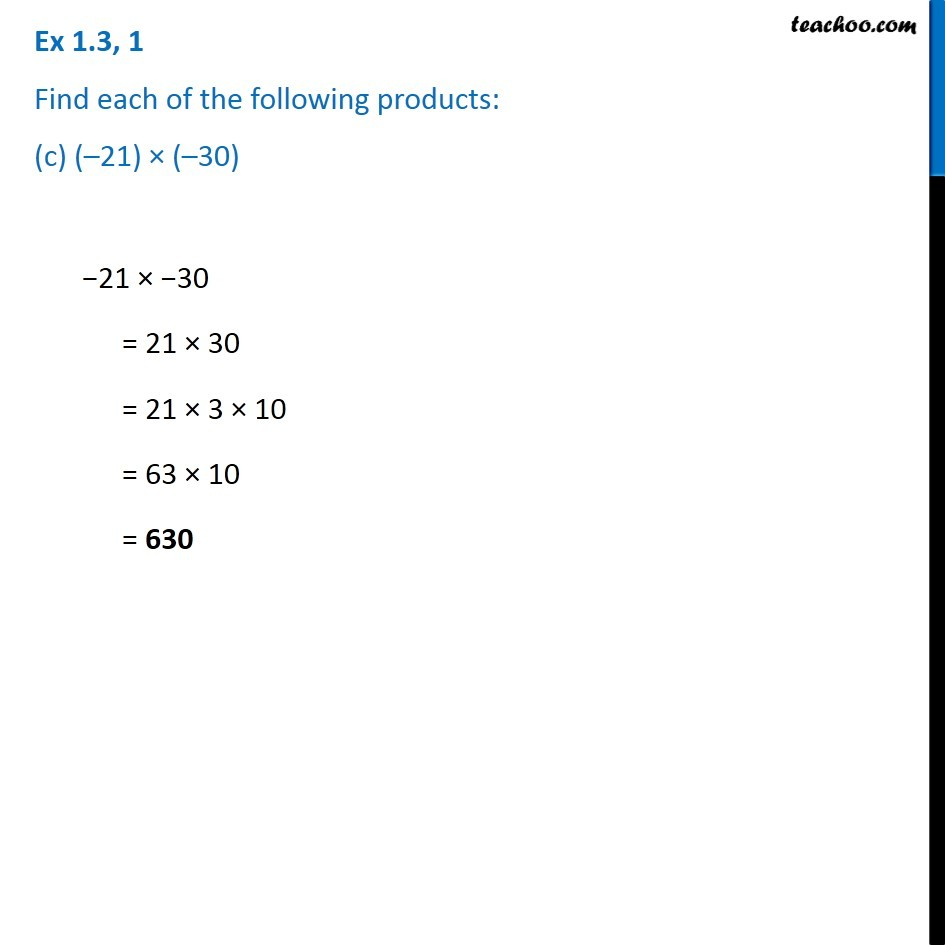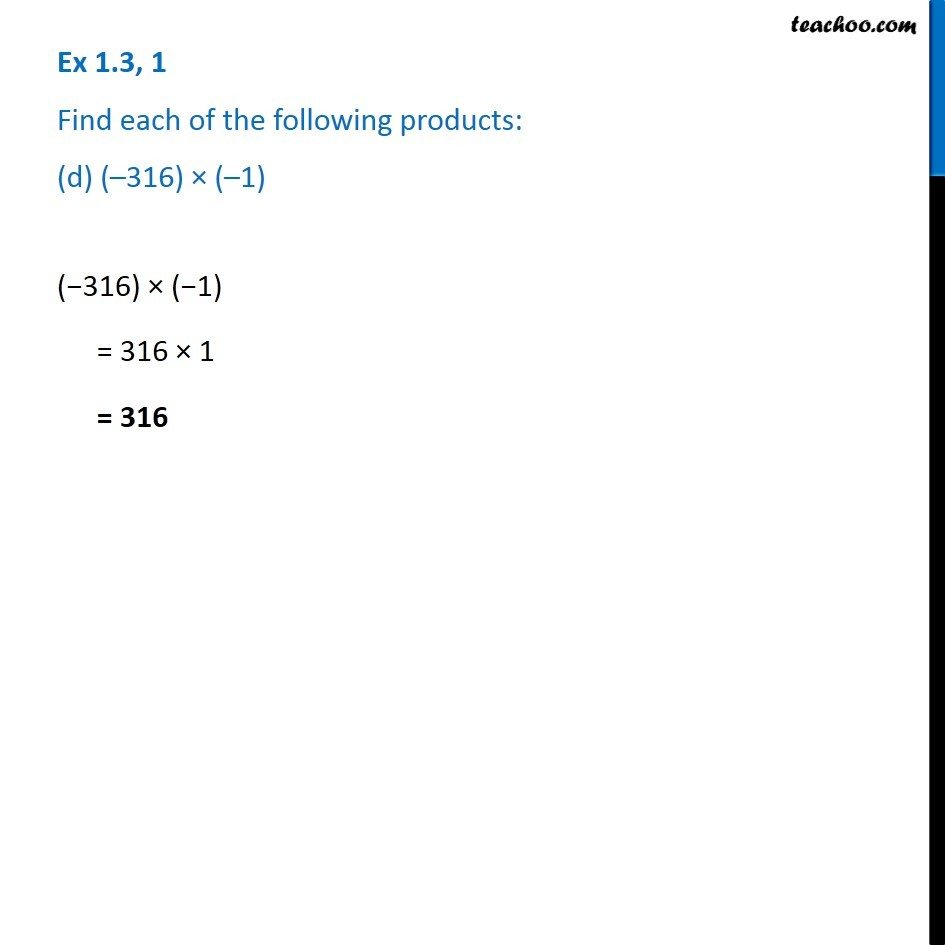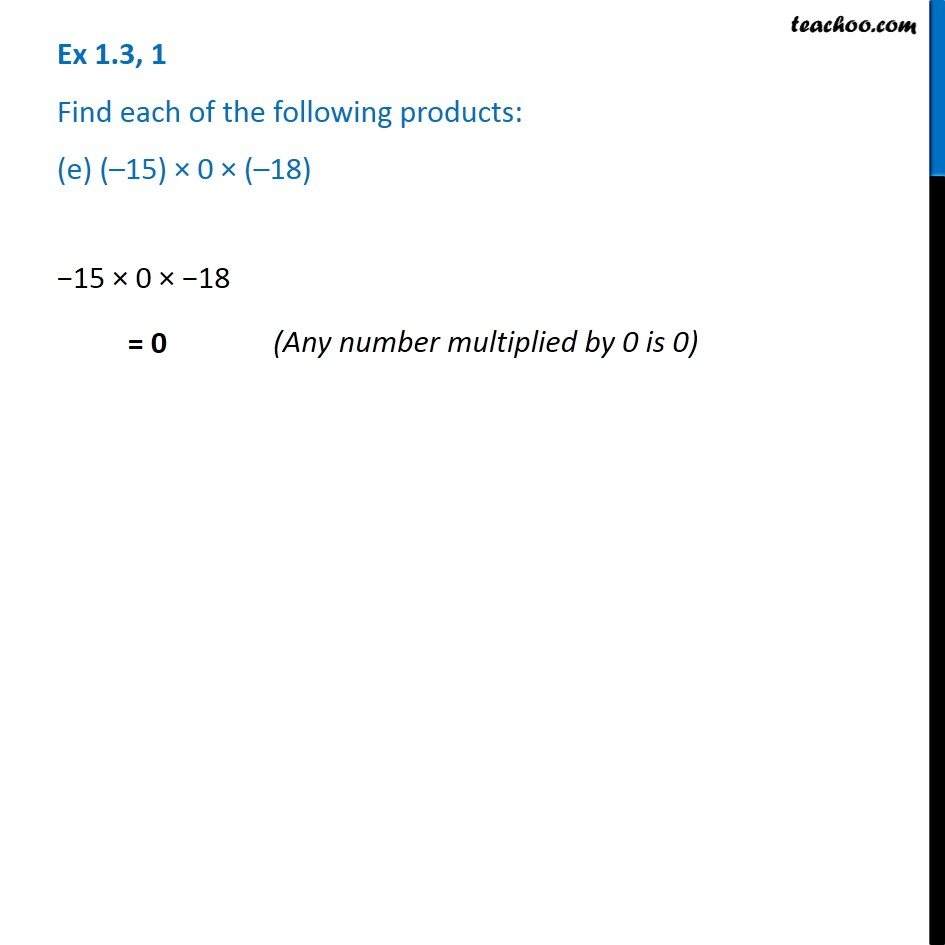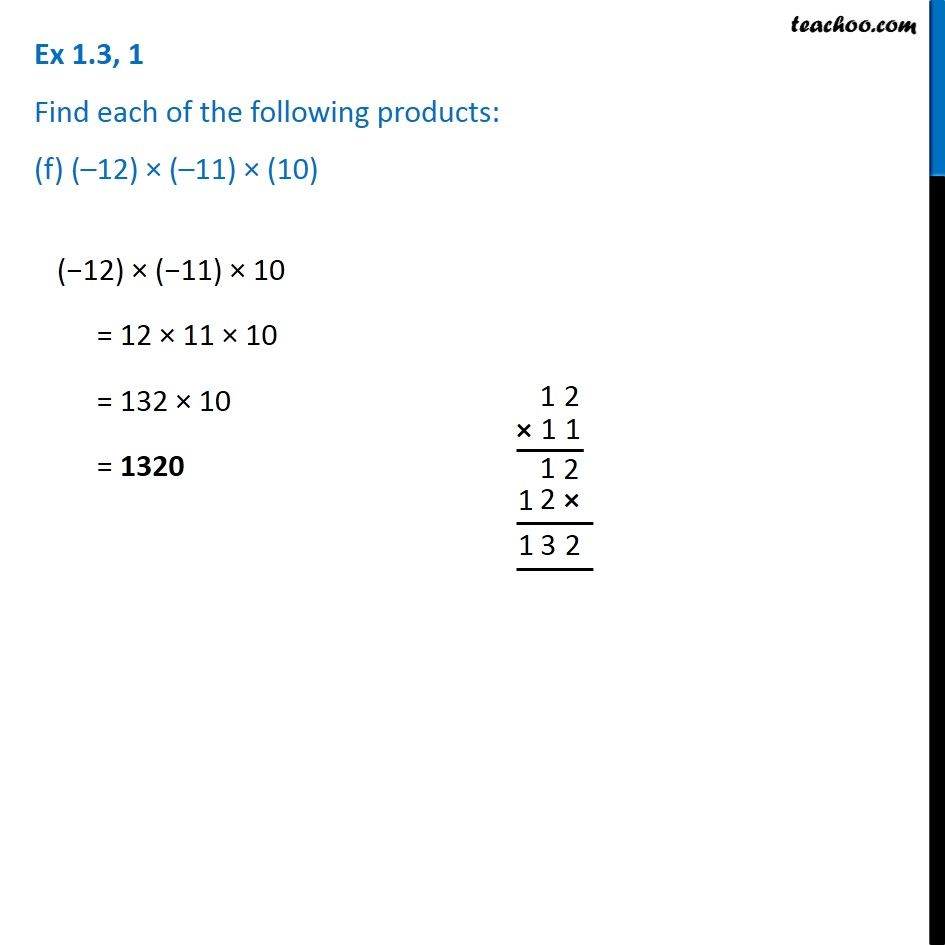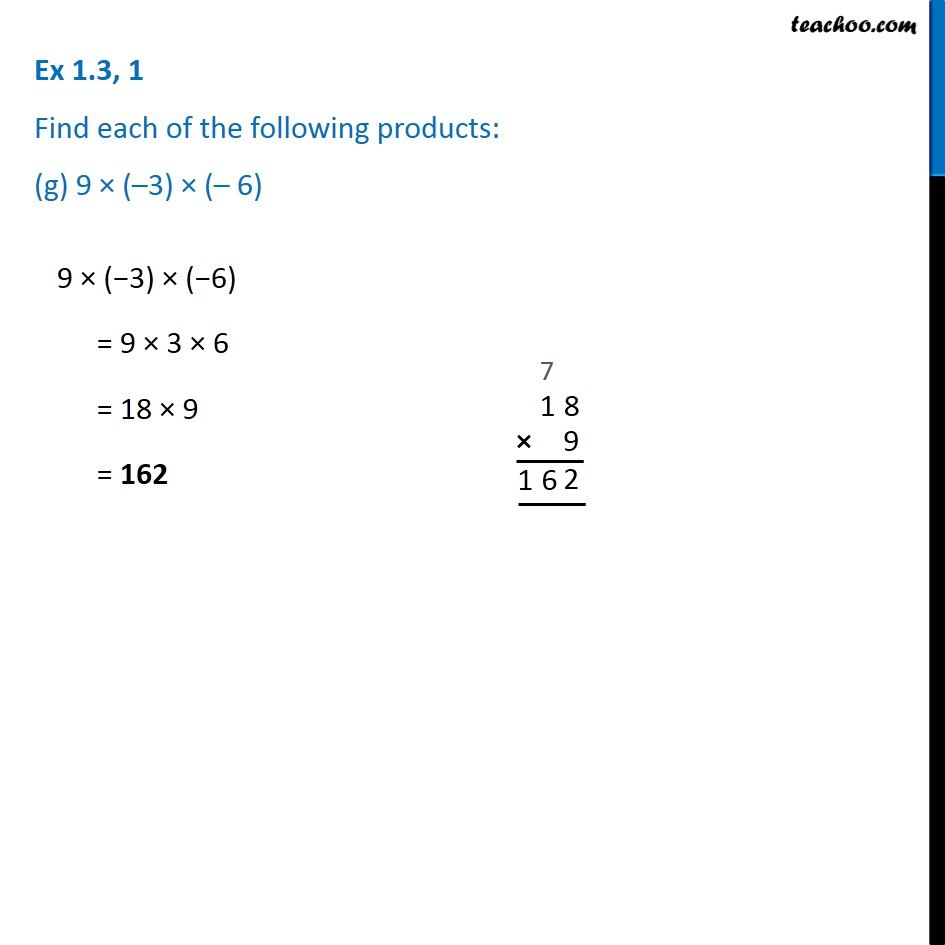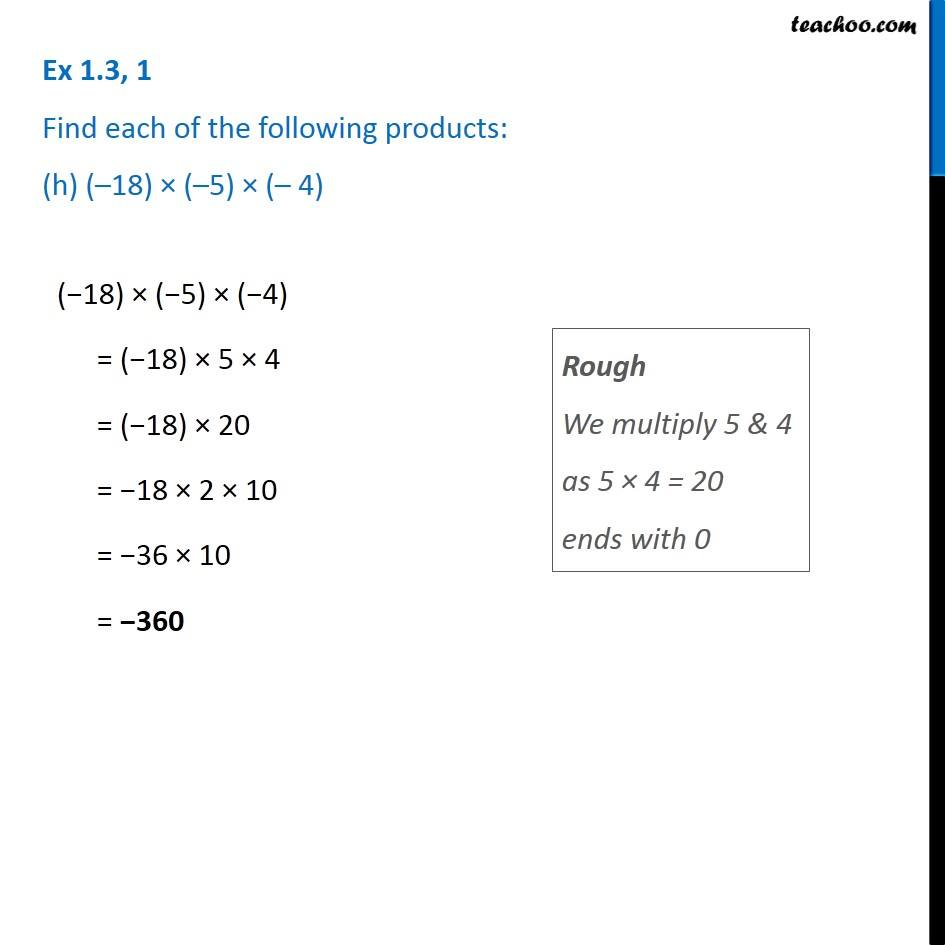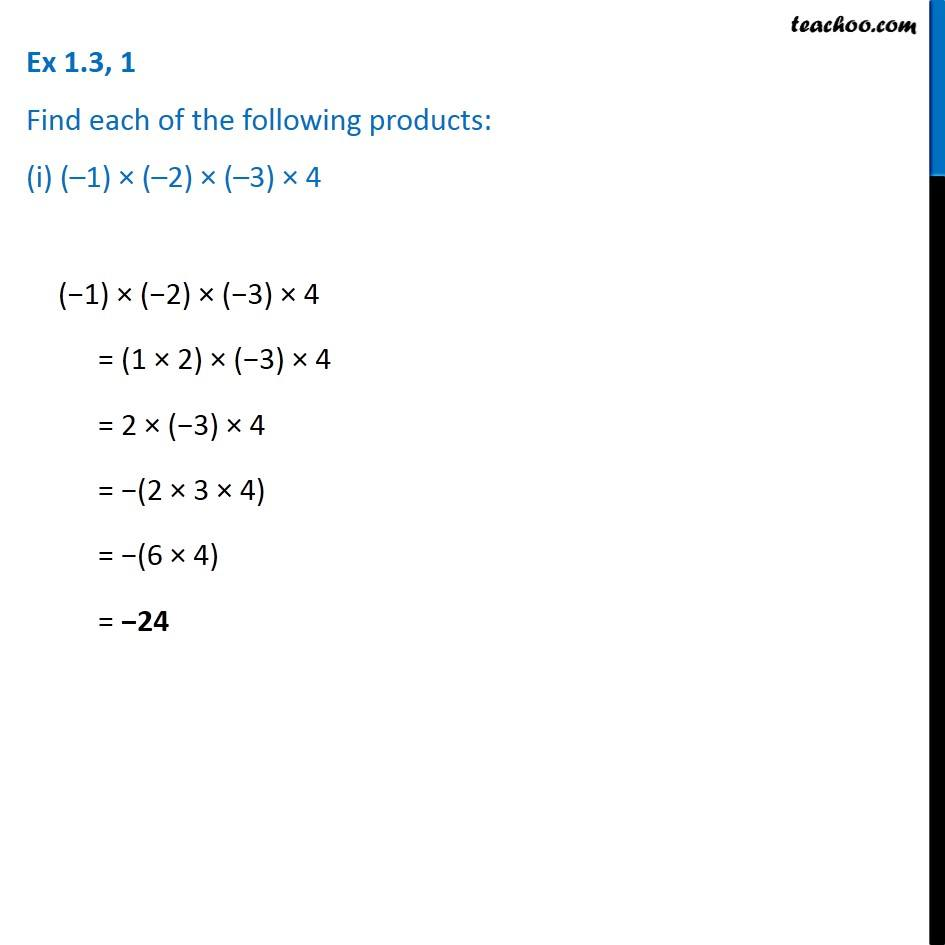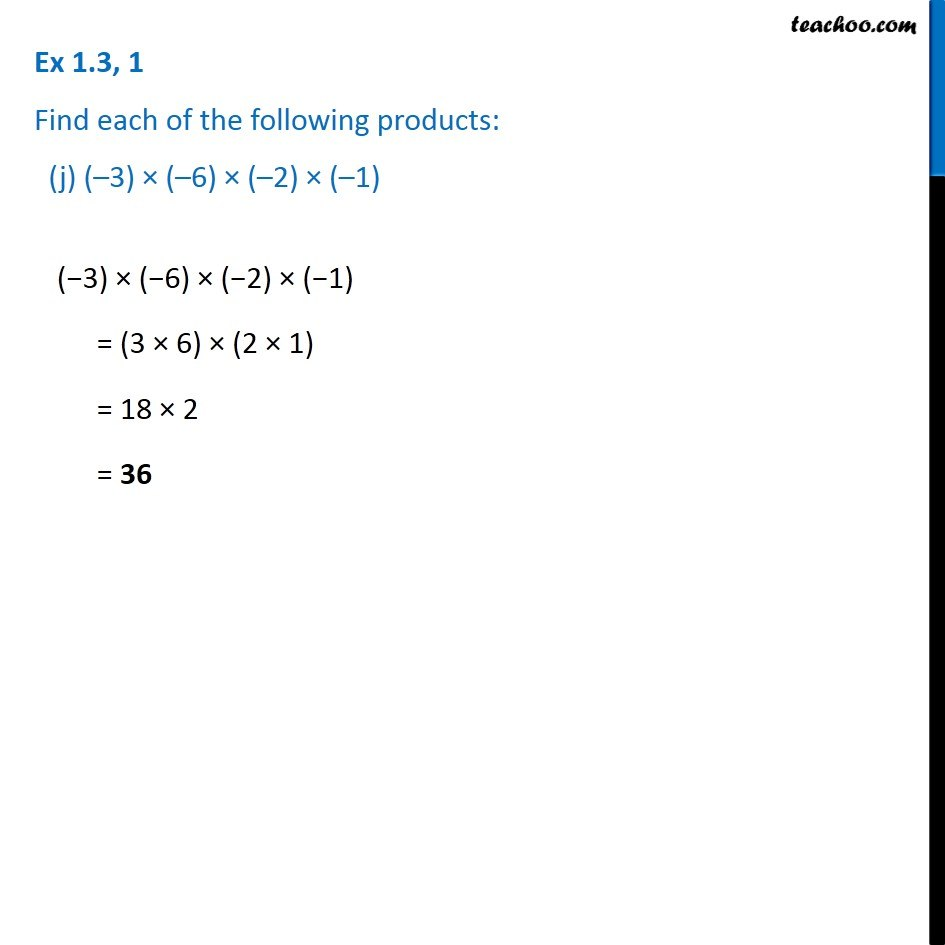1. Chapter 1 Class 7 Integers
2. Concept wise
3. Multiplication of Integers

Transcript

Ex 1.3, 1 Find each of the following products: (a) 3 × (–1) 3 × (−1) = −3 Ex 1.3, 1 Find each of the following products: (b) (–1) × 225 (−1) × 225 = −225 Ex 1.3, 1 Find each of the following products: (c) (–21) × (–30) −21 × −30 = 21 × 30 = 21 × 3 × 10 = 63 × 10 = 630 Ex 1.3, 1 Find each of the following products: (d) (–316) × (–1) (−316) × (−1) = 316 × 1 = 316 Ex 1.3, 1 Find each of the following products: (e) (–15) × 0 × (–18) −15 × 0 × −18 = 0 (Any number multiplied by 0 is 0) Ex 1.3, 1 Find each of the following products: (f) (–12) × (–11) × (10) (−12) × (−11) × 10 = 12 × 11 × 10 = 132 × 10 = 1320 Ex 1.3, 1 Find each of the following products: (g) 9 × (–3) × (– 6) 9 × (−3) × (−6) = 9 × 3 × 6 = 18 × 9 = 162 Ex 1.3, 1 Find each of the following products: (h) (–18) × (–5) × (– 4) (−18) × (−5) × (−4) = (−18) × 5 × 4 = (−18) × 20 = −18 × 2 × 10 = −36 × 10 = −360 Ex 1.3, 1 Find each of the following products: (i) (–1) × (–2) × (–3) × 4 (−1) × (−2) × (−3) × 4 = (1 × 2) × (−3) × 4 = 2 × (−3) × 4 = −(2 × 3 × 4) = −(6 × 4) = −24 Ex 1.3, 1 Find each of the following products: (j) (–3) × (–6) × (–2) × (–1) (−3) × (−6) × (−2) × (−1) = (3 × 6) × (2 × 1) = 18 × 2 = 36